August 15, 2016

# R graphics

There are many ways to make graphics in R.

• base R
• lattice graphics
• ggplot2

# ggplot2

ggplot2 is an R package by Hadley Wickham that lets you make beautiful R graphics (relatively) easily.

It’s part of the tidyverse, which I recommend everyone get to know (dplyr, stringr, lubridate, broom… and many more).

The name ggplot2 refers to a famous book on data visualization theory called The Grammar of Graphics.

# Getting started

First, we need to install and load the package,

``````#install.packages("ggplot2")
library(ggplot2)``````

# Diamonds data

To start, I’m going to use the diamonds data that comes with ggplot2,

``str(diamonds)``
``````## Classes 'tbl_df', 'tbl' and 'data.frame':    53940 obs. of  10 variables:
##  \$ carat  : num  0.23 0.21 0.23 0.29 0.31 0.24 0.24 0.26 0.22 0.23 ...
##  \$ cut    : Ord.factor w/ 5 levels "Fair"<"Good"<..: 5 4 2 4 2 3 3 3 1 3 ...
##  \$ color  : Ord.factor w/ 7 levels "D"<"E"<"F"<"G"<..: 2 2 2 6 7 7 6 5 2 5 ...
##  \$ clarity: Ord.factor w/ 8 levels "I1"<"SI2"<"SI1"<..: 2 3 5 4 2 6 7 3 4 5 ...
##  \$ depth  : num  61.5 59.8 56.9 62.4 63.3 62.8 62.3 61.9 65.1 59.4 ...
##  \$ table  : num  55 61 65 58 58 57 57 55 61 61 ...
##  \$ price  : int  326 326 327 334 335 336 336 337 337 338 ...
##  \$ x      : num  3.95 3.89 4.05 4.2 4.34 3.94 3.95 4.07 3.87 4 ...
##  \$ y      : num  3.98 3.84 4.07 4.23 4.35 3.96 3.98 4.11 3.78 4.05 ...
##  \$ z      : num  2.43 2.31 2.31 2.63 2.75 2.48 2.47 2.53 2.49 2.39 ...``````

# ATUS data

You guys are going to get to follow along with the diamonds data, but also try things out on the American Time Use Survey data.

I’ve given you this data here. You can download this the point-and-click way, or do it programmatically.

``````library(RCurl)
htmlData <- getURL("https://github.com/AmeliaMN/SummerDataViz/blob/master/ggplot2_intro/atus.csv")

# qplot()– the easy way out

``qplot(carat, data=diamonds)``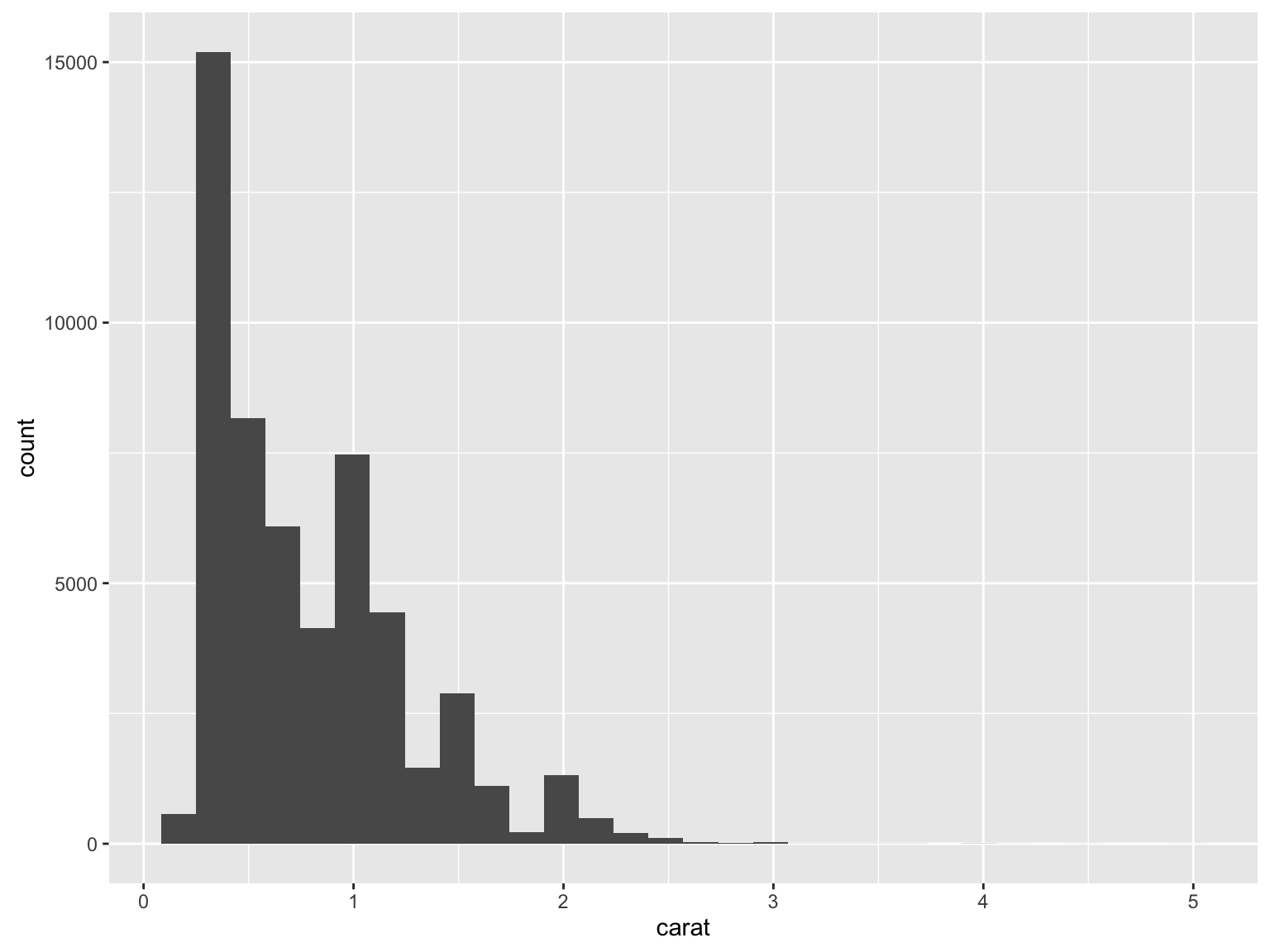# ggplot2 syntax

``qplot(carat, data=diamonds)``

qplot() performs similar functionality to the base R graphics function plot(). But it aleady might seem a little different, because we’re not using the \$ operator.

Instead, you’re listing the name of the variable(s) and then telling R where to “look” for that variable with “data=”. This is like what we do when modeling using functions like lm().

# More qplot()

``qplot(clarity, fill=cut, data=diamonds)``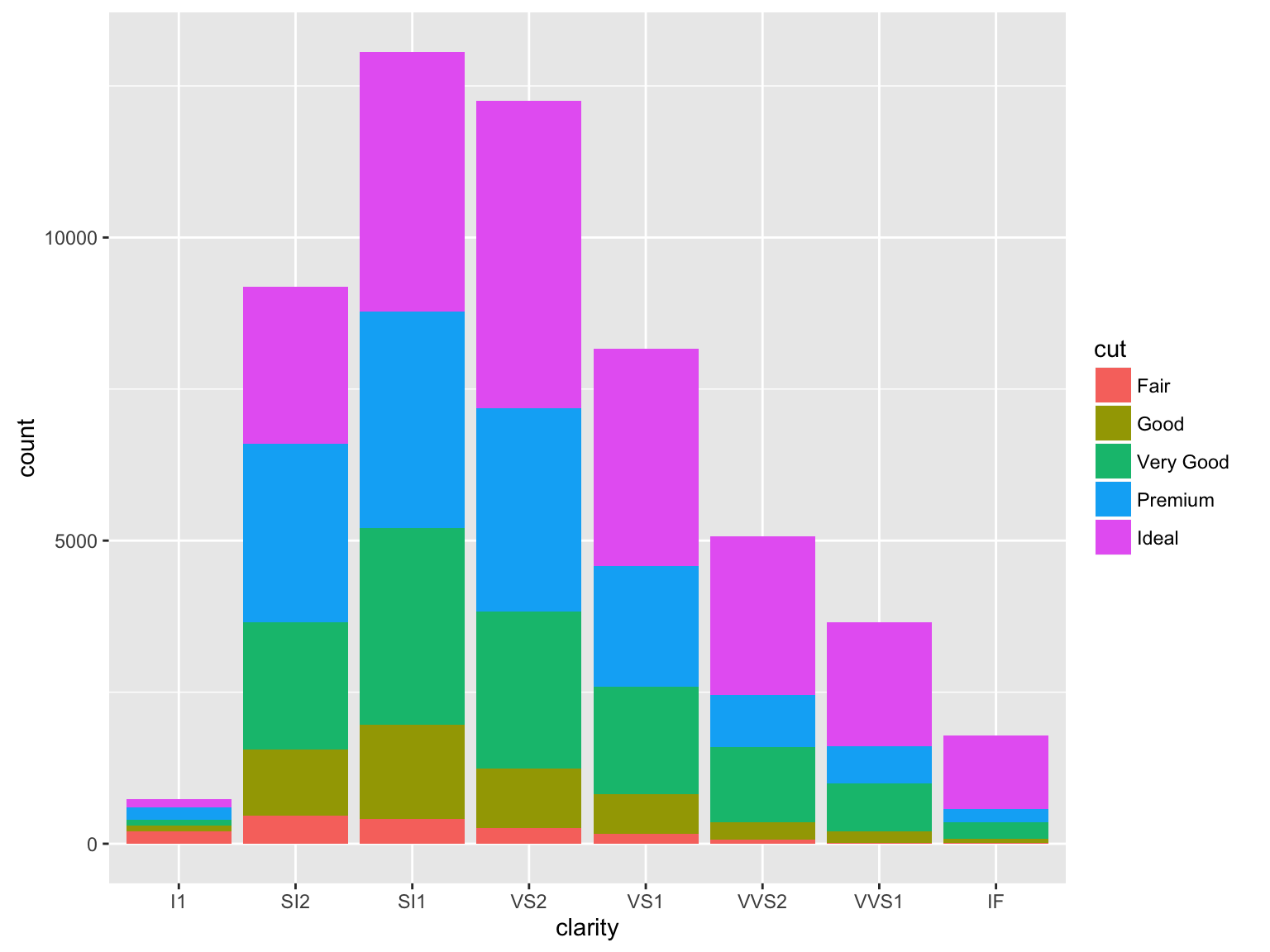# ggplot()

But, in order to really harness the power of ggplot2 you need to use the more general ggplot() command. The idea of the package is you can “layer” pieces on top of a plot to build it up over time.

You always need to use a ggplot() call to initialize the plot. I usually put my dataset in here, and at least some of my “aesthetics.” But, one of the things that can make ggplot2 tough to understand is that there are no hard and fast rules.

``p1 <- ggplot(aes(x=clarity, fill=cut), data=diamonds)``

If you try to show p1 at this point, you will get “Error: No layers in plot.” This is because we haven’t given it any geometric objects yet.

# geoms

In order to get a plot to work, you need to use “geoms” (geometric objects) to specify the way you want your variables mapped to graphical parameters.

``p1 + geom_bar()``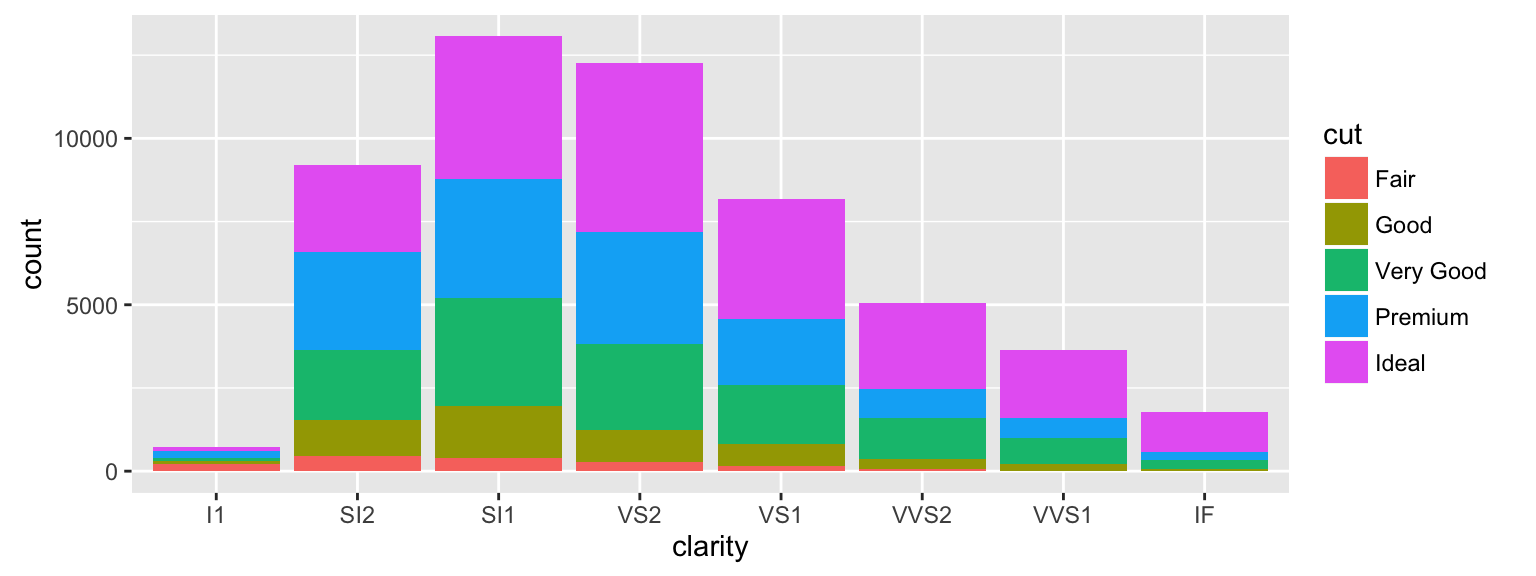# geoms have options

``p1 + geom_bar(position="dodge")``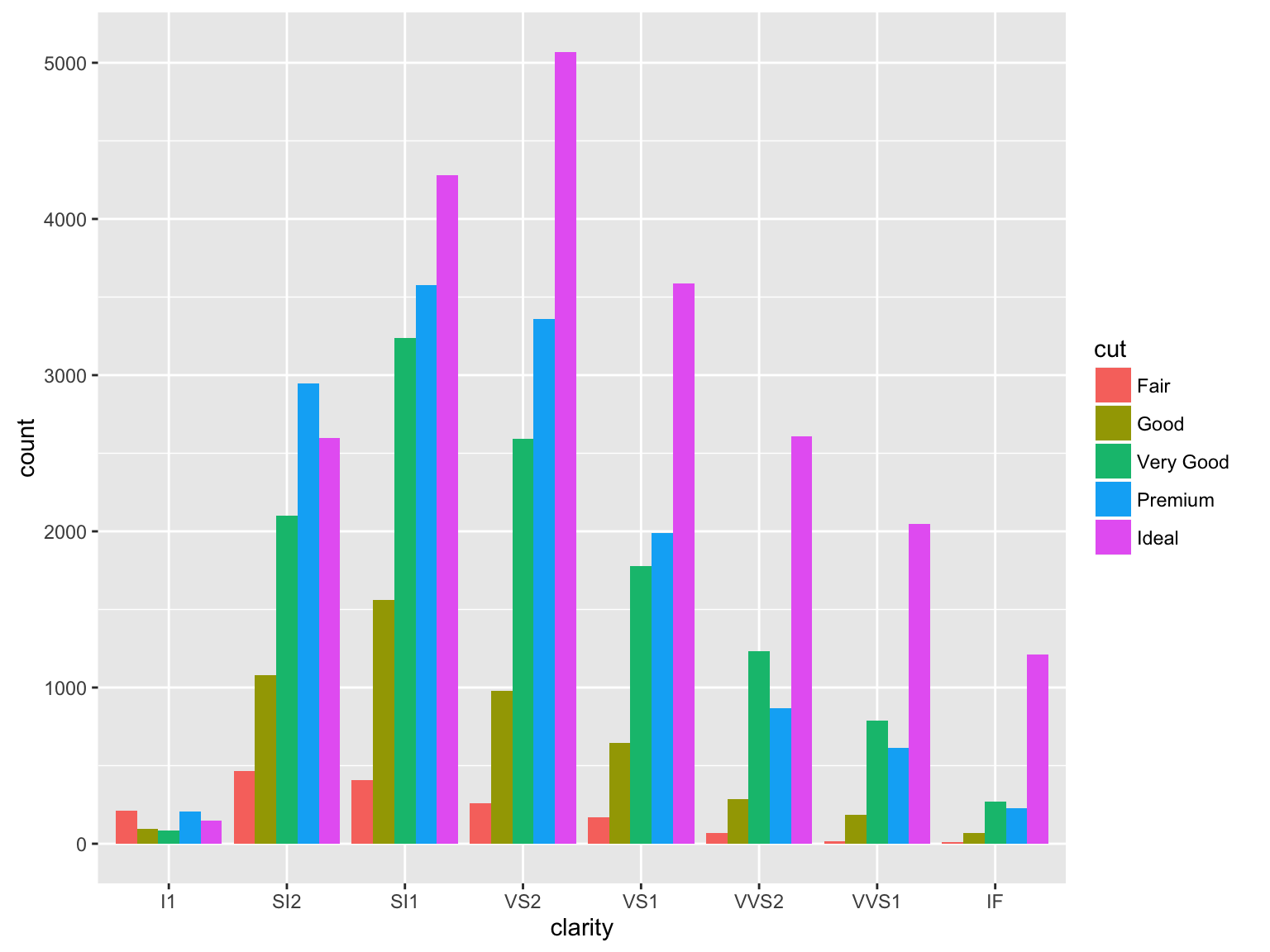# Lots of options

``p1 + geom_bar(position="fill")``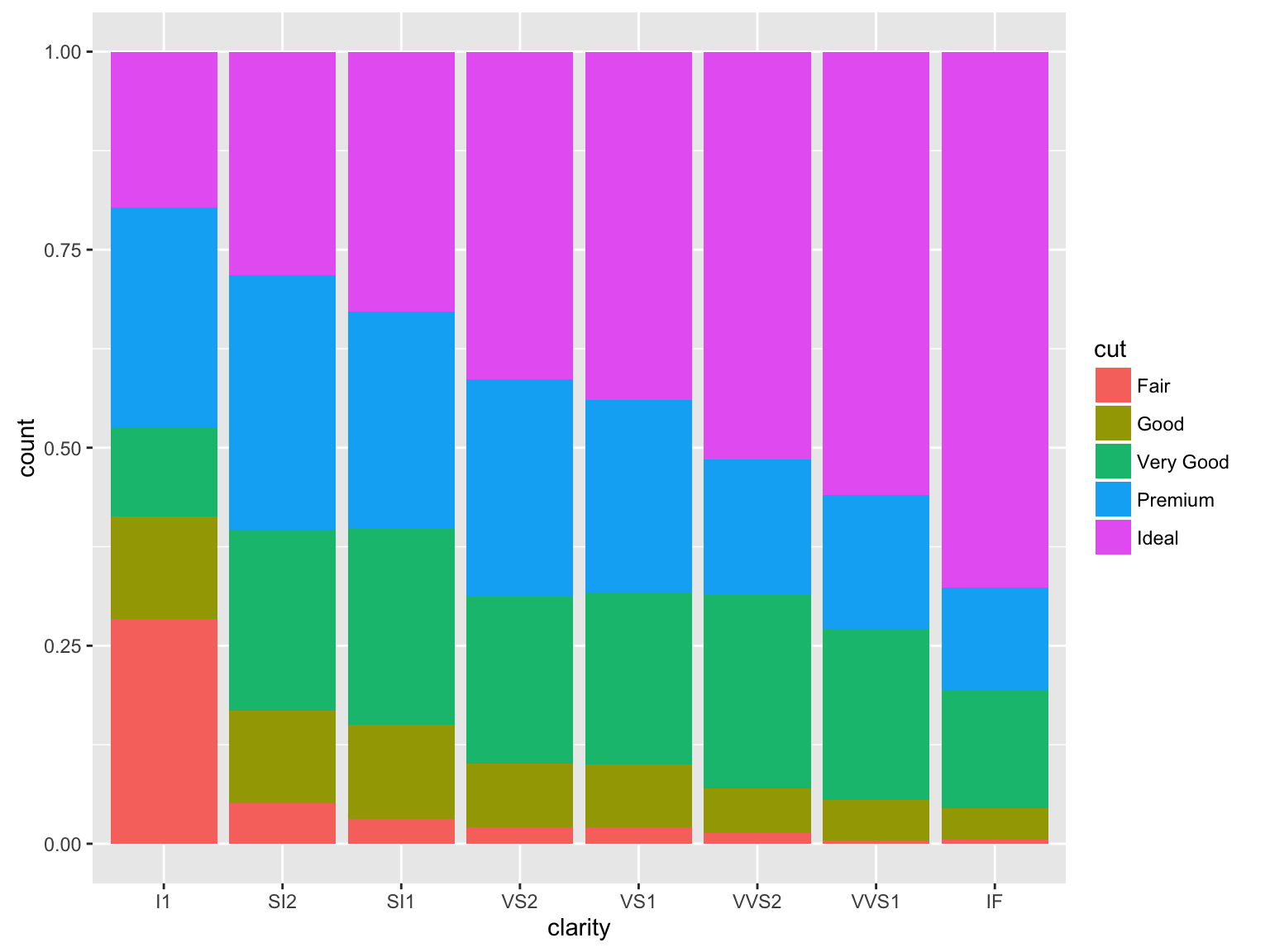# Two variables

``````p2 <- ggplot(aes(x=carat, y=depth), data=diamonds)
p2 + geom_point()``````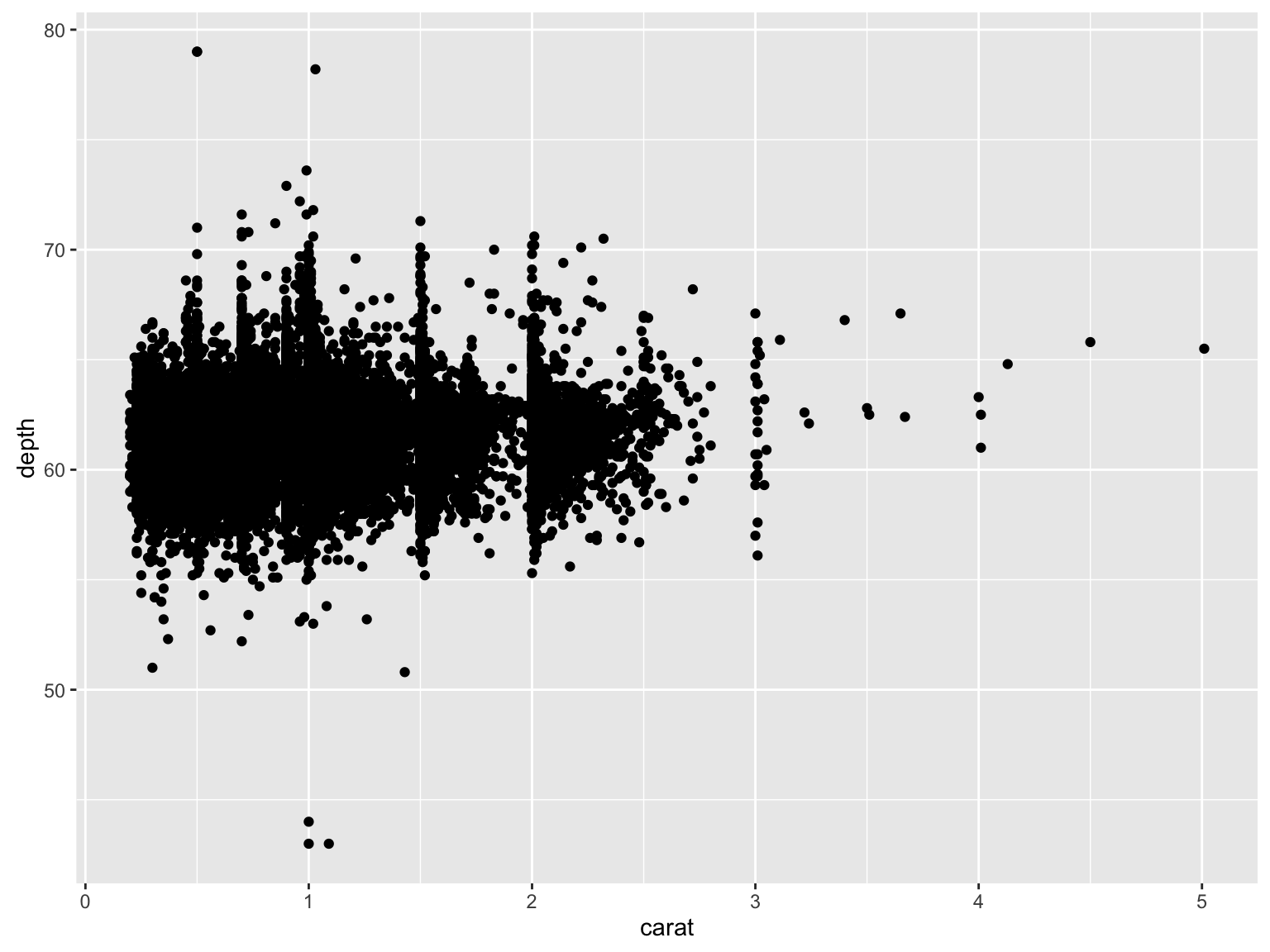# Same data, different geom

``p2 + geom_bin2d()``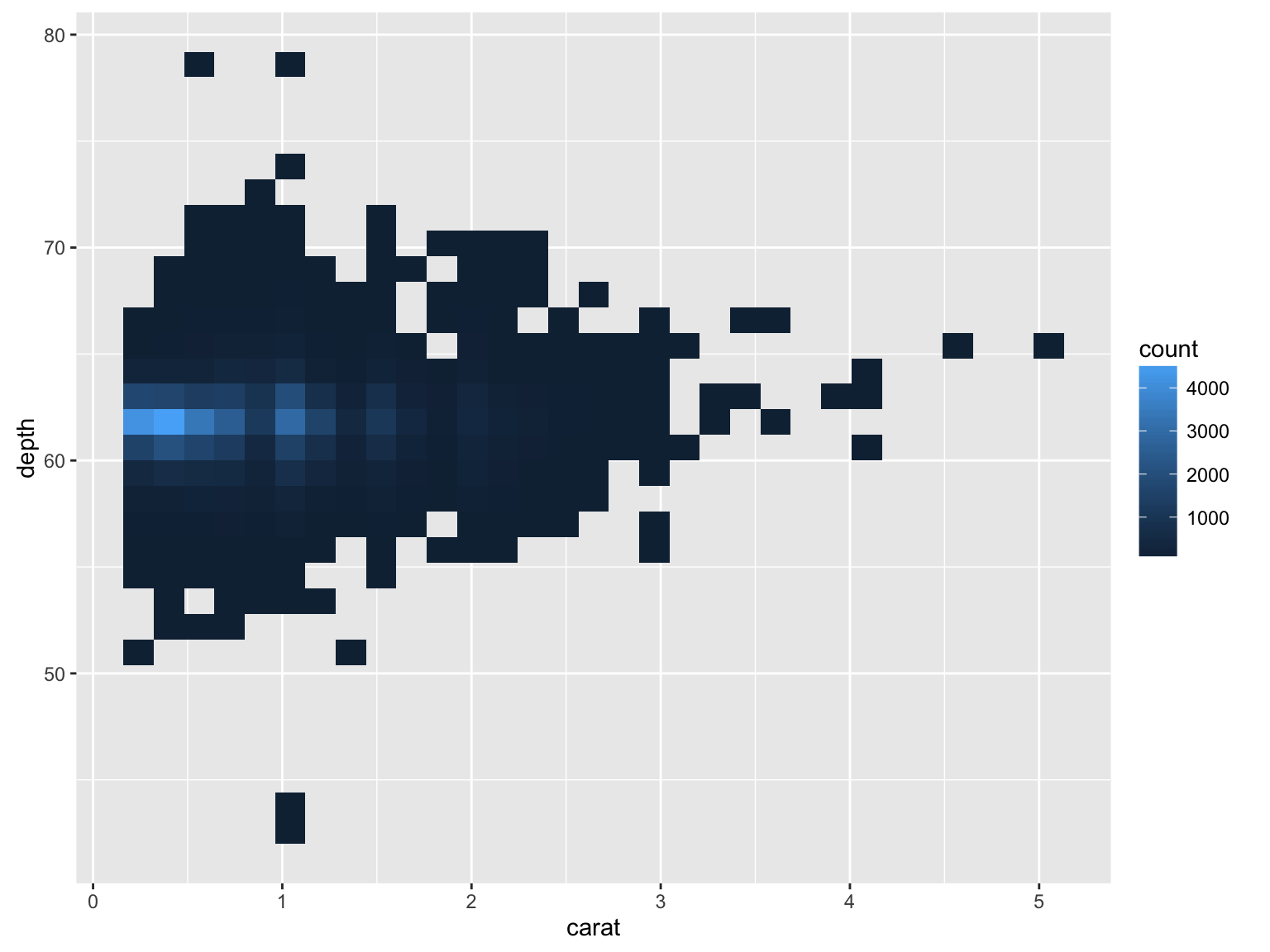# Saving your work (or not)

Notice that I’m not saving these geom layers– I’m just running

p2 + [something]

to see what happens. But, I can save the new version to start building up my plot,

``p2 <- p2 + geom_bin2d()``

# Better labels

``````p2 <- p2 + xlab("Carat") + ylab("Depth") +
guides(fill=guide_legend(title="Number of diamonds"))
p2``````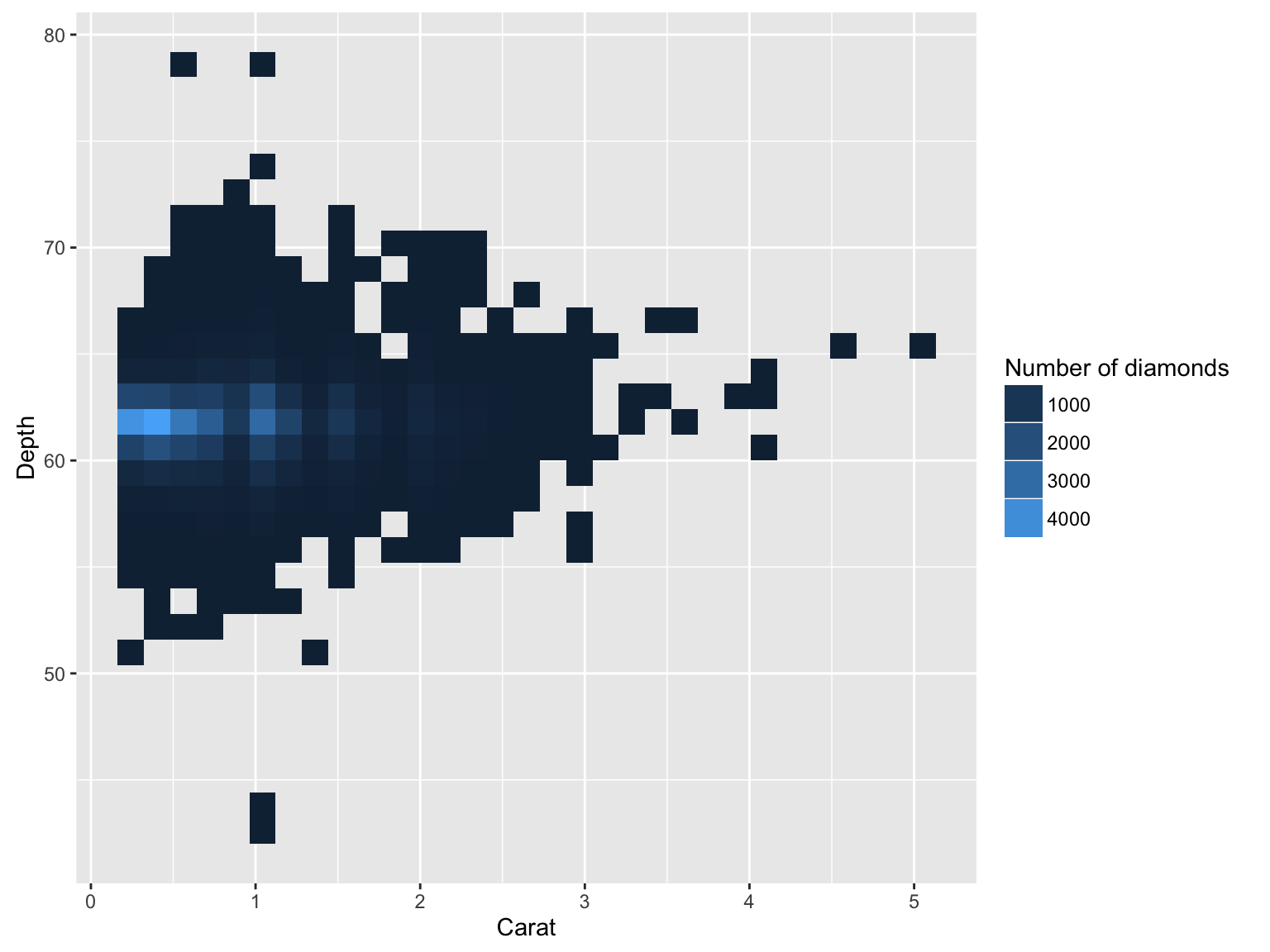# Different breaks

``p2 + scale_fill_continuous(breaks=c(1500, 2500, 3500,4500))``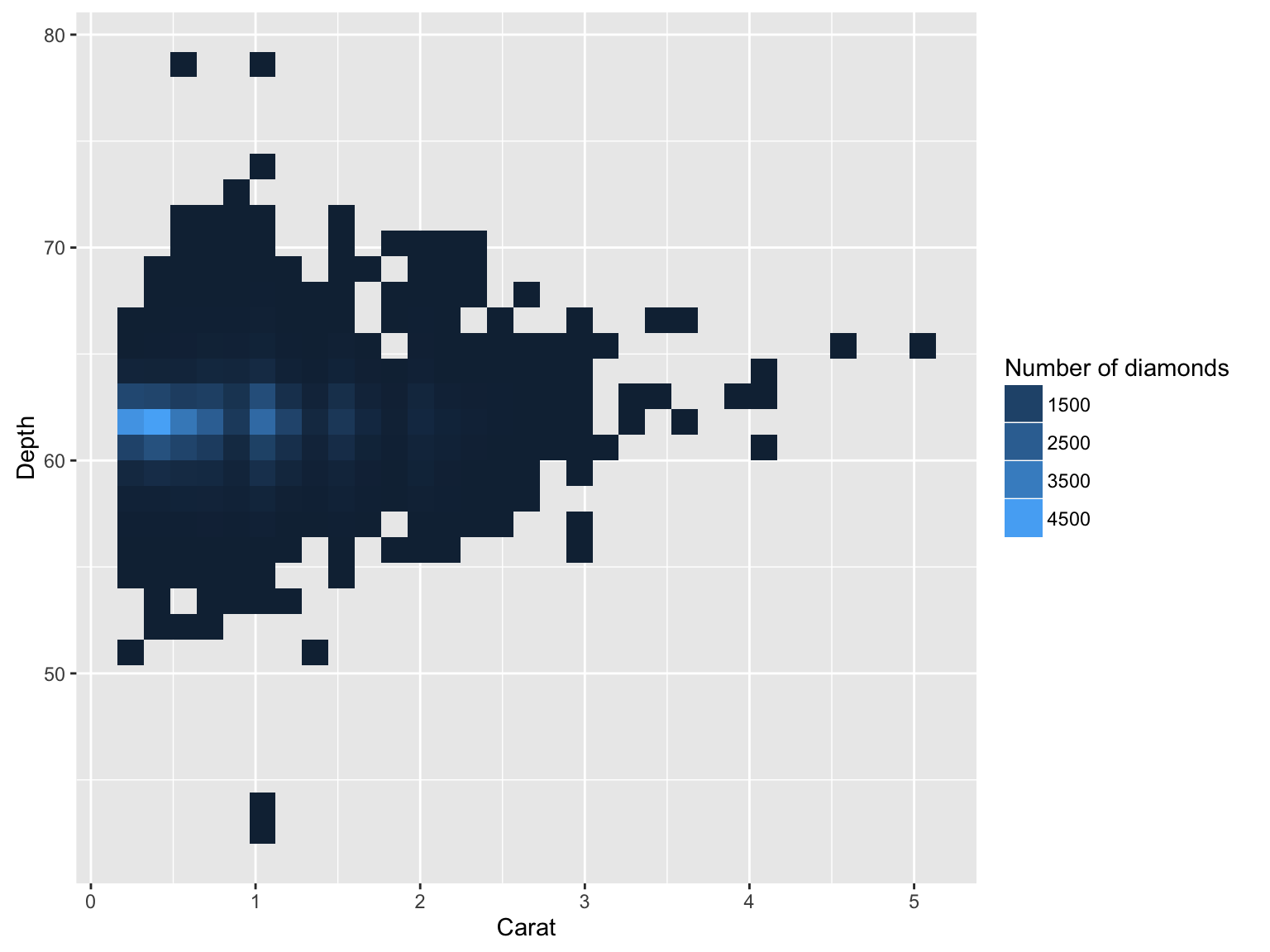# Log scale

``p2 + scale_fill_continuous(trans="log")``